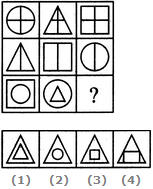# Non Verbal Reasoning - Figure Matrix - Discussion

### Discussion :: Figure Matrix - Section 2 (Q.No.6)

In each of the following questions, find out which of the answer figures (1), (2), (3) and (4) completes the figure matrix ?

6.

Select a suitable figure from the four alternatives that would complete the figure matrix.[A]. 1 [B]. 2 [C]. 3 [D]. 4

Explanation:

In each row, the triangle follows the circle, the square follows the triangle and the circle follows the square. In case of the third row, the above rule exists for the inner as well as the outer elements.

 Dharma said: (Oct 9, 2018) I am not understanding this. Please explain the answer.

 Mikayla said: (Dec 4, 2018) Anyone explain the solution clearly.

 Vanshika said: (May 24, 2019) How possible? Please explain in detail.

 Aadil.Nabi said: (Jul 5, 2019) In first row, each figure has four divisions. In the second row, each figure has two divisions. In the third row, each figure encloses different figure without touching. So part (3) will be answer. Part (2) will not be the answer because the circle is already enclosed.

 Sai Rithwik said: (Sep 9, 2019) I can't understand it. Please, anyone, explain it.